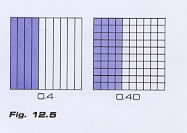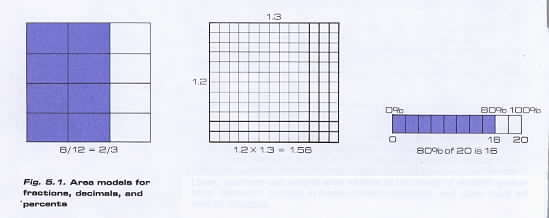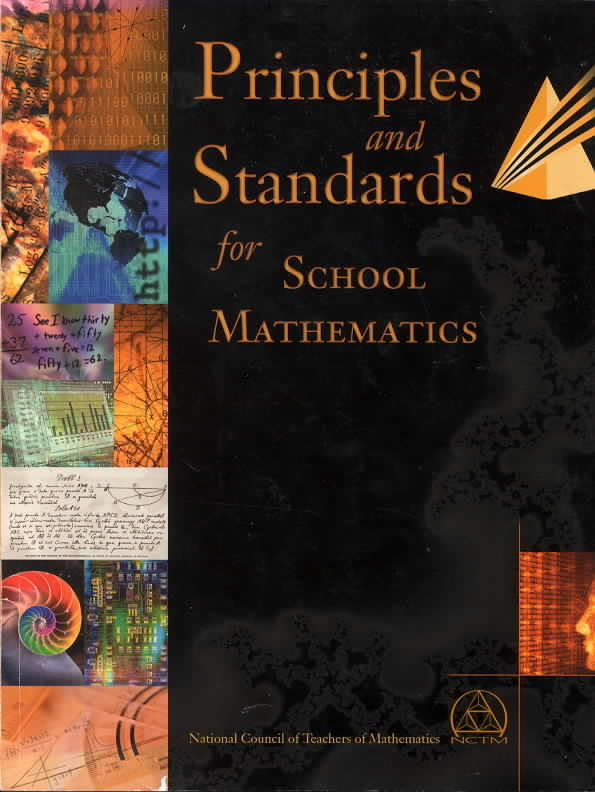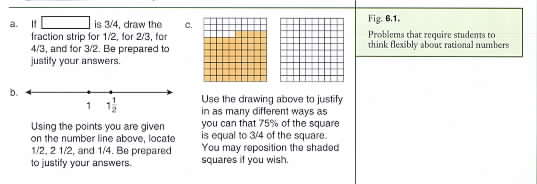Home
 What NCTM's 1989 & 2000 Standards Say About Teaching DecimalsStatements from NCTM's 1989 Standards on Teaching Decimals The K-4 instruction should help students understand fractions and decimals explore their relationship and build initial concepts about order and equivalence. Because evidence suggests that children construct these ideas slowly it is crucial that teachers use physical materials diagrams and real-world situations in conjunction with ongoing efforts to relate their learning experiences to oral language and symbols. This K-4 emphasis on basic ideas will reduce the amount of time currently spent in the upper grades in correcting students’ misconceptions and procedural difficulties. In grades K-4 children begin to encounter decimals in many situations – with calculators and metric measures in tables of data and in such daily activities as using a digital stopwatch. Thus the curriculum needs to emphasize the development of decimal concepts. The approach to decimals should be similar to work with fractions namely placing a strong and continued emphasis on models and oral language and then connecting this work with symbols. This is necessary if students are to make sense of decimals and use them insightfully. Exploring ideas of tenths and hundredths with models can include preliminary work with equivalent decimals (fig. 12.5) counting sequences the comparing and ordering of decimals and addition and subtraction.Decimal instruction should include informal experiences that relate fractions to decimals so that students begin to establish connections between the two systems. For example if students recognize that 1/2 is the same amount as 0.5 they can use this relationship to determine that 0.4 and 0.45 are a little less than 1/2 and that 0.6 and 0.57 are a little more than 1/2. Such activities help children develop number sense for decimals. To provide students with a lasting sense of number and number relationships learning should be grounded in experience related to aspects of everyday life or to the use of concrete materials designed to reflect underlying mathematical ideas. Students should encounter number lines area models and graphs as well as representations of numbers that appear on calculators and computers (e.g. forms of scientific notation). Students should learn to identify equivalent forms of a number and understand why a particular representation is useful in a given setting. Understanding multiple representations for numbers is a crucial precursor to solving many of the problems students encounter. Toward this end students can represent fractions decimals and percents in a variety of meaningful situations thereby learning to move flexibly among concrete pictorial and abstract representations. Students should understand these numbers and their representations the relationships among them and the advantages and disadvantages of each. Exponents and scientific notation give further examples of the elegance and complexities of concise notation. Numbers like 2 ^ 30 in the denominator of a fraction in a probability problem ^10 as a factor in a population growth problem x 10 ^ -8 in a science problem and \$3.46 million in a newspaper article are approximate representations each of which is best suited to its own context. Calculators and computers can display forms like 7.23471 07 thus requiring that students learn other forms of scientific notation. Students also should recognize the difficulties inherent in various representations such as the expression of 1/3 as a percent x 10 ^ -9 as an ordinary decimal or 5.999999999 on a calculator as 6. They also need to grasp some sense of the "infinite" quality of decimals. Area models are especially helpful in visualizing numerical ideas from a geometric point of view. For example area models can be used to show that 8/12 is equivalent to 2/3 that 1.2 x 1.3 = 1.56 and that 80% of 20 is 16. see figure 5.1. Later students can extend area models to the study of algebra probability dimension analysis in measurement situations and other more advanced subjects.Concrete materials and representational models (pictures) should be used to continue the study of place value initiated in grade K-4. For example students should see that the model in figure 5.2 represents 346 or 3.46 according to whether a small or a large square represents a unit.Once students are familiar with a particular type of representation it can be generalized and the equivalence of different representations for the same number can be established. This process should be approached conceptually before the computational techniques that convert one to another are developed. .Statements from NCTM's 2000 Standards on Teaching Decimals Calculators should be available at appropriate times as computational tools particularly when many or cumbersome computations are needed to solve problems. However when teachers are working with students on developing computational algorithms the calculator should be set aside to allow this focus. Today the calculator is a commonly used computational tool outside the classroom and the environment inside the classroom should reflect this reality. Representing numbers with various physical materials should be a major part of mathematics instruction in the elementary school grades. By the middle grades students should understand that numbers can be represented in various ways so that they see that 1/4 and 0.25 are all different names for the same number. Students’ understanding and ability to reason will grow as they represent fractions and decimals with physical materials and on number lines and as they learn to generate equivalent representations of fractions and decimals. Experience suggests that in classes focused on the development and discussion of strategies various "standard" algorithms either arise naturally or can be introduced by the teacher as appropriate. The point is that students must become fluent in arithmetic computation – they must have efficient and accurate methods that are supported by an understanding of numbers and operations. "Standard" algorithms for arithmetic computation are one means of achieving this fluency. Concrete models can help students represent numbers and develop number sense; they can also help bring meaning to students’ use of written symbols and can be useful in building place-value concepts. But using materials especially in a rote manner does not ensure understanding. Teachers should try to uncover students’ thinking as they work with concrete materials by asking questions that elicit students’ thinking and reasoning. In this way teachers can watch for students’ misconceptions such as interpreting the 2 tens and 3 ones in figure 4.1c merely as five objects. Teachers should also choose interesting tasks that engage students in mathematical thinking and reasoning which builds their understanding of numbers and relationships among numbers. Students in these grades should use models and other strategies to represent and study decimal numbers. For example they should count by tenths (one-tenth two-tenths three-tenths …) verbally or use a calculator to link and relate whole numbers with decimal numbers. As students continue to count orally from nine-tenths to ten-tenths to eleven-tenths and see the display change from 0.9 to 1.0 to 1.1 they see that ten-tenths is the same as one and also how it relates to 0.9 and 1.1. Through a variety of activities they should understand that a fraction such as 1/2 is equivalent to 5/10 and that it has a decimal representation (0.5). As they encounter a new meaning of a fraction – as a quotient of two whole numbers (1/2 = 1 ÷ 2 = 0.5) – they can also see another way to arrive at this equivalence. By using the calculator to carry out the indicated division of familiar fractions like 1/4 and 3/4 they can learn common fraction-decimal equivalents. They can also learn that some fractions can be expressed as terminating decimals but others cannot. Students should understand the meaning of a percent as part of a whole and use common percents such as 10 percent percent or 50 percent as benchmarks in interpreting situations they encounter. For example if a label indicates that 36 percent of a product is water students can think of this as about a third of the product. By studying fractions decimals and percents simultaneously students can learn to move among equivalent forms choosing and using an appropriate and convenient form to solve problems and express quantities. Estimation serves as an important companion to computation. It provides a tool for judging the reasonableness of calculator mental and paper-and-pencil computations. However being able to compute exact answers does not automatically lead to an ability to estimate or judge the reasonableness of answers as Reys and Yang (1998) found in their work with sixth and eighth graders. Students in grades 3-5 will need to be encouraged to routinely reflect on the size of an anticipated solution. The study of rational numbers in the middle grades should build on students’ prior knowledge of whole-number concepts and skills and their encounters with fractions decimals and percents in lower grades and in everyday life. In the middle grades students should become facile in working with fractions decimals and percents. Teachers can help students deepen their understanding of rational numbers by presenting problems such as those in figure 6.1 that call for flexible thinking. .At the heart of flexibility in working with rational numbers is a solid understanding of different representations for fractions decimals and percents. In grades 3-5 students should have learned to generate and recognize equivalent forms of fractions decimals and percents at least in some simple cases. In the middle grades students should build on and extend this experience to become facile in using fractions decimals and percents meaningfully. Students can develop a deep understanding of rational numbers through experiences with a variety of models such as fraction strips number lines x 10 grids area models and objects. These models offer students concrete representations of abstract ideas and support students’ meaningful use of representations and their flexible movement among them to solve problems. The foundation of students’ work with decimal numbers must be an understanding of whole numbers and place value. In grades 3-5 students should have learned to think of decimal numbers as a natural extension of the base-ten place-value system to represent quantities less than 1. In grades 6-8 they should also understand decimals as fractions whose denominators are powers of 10. The absence of a solid conceptual foundation can greatly hinder students. Without a solid conceptual foundation students often think about decimal numbers incorrectly; they may for example think that 3.75 is larger than 3.8 because 75 is more than 8 (Resnick et al. 1989). Students also need to interpret decimal numbers as they appear on calculator screens where they may be truncated or rounded. Percents which can be thought about in ways that combine aspects of both fractions and decimals offer students another useful form of rational number. Percents are particularly useful when comparing fractional parts of sets or numbers of unequal size and they are also frequently encountered in problem-solving situations that arise in everyday life. As with fractions and decimals conceptual difficulties need to be carefully addressed in instruction. In particular percents less than 1 percent and greater than 100 percent are often challenging and most students are likely to benefit from frequent encounters with problems involving percents of these magnitudes in order to develop a solid understanding. Students should develop a sense of the magnitude of very large numbers (millions and billions) and become proficient at reading and representing them. For example they should recognize and represent 2 300 000 000 as 2.3 x 10 ^ 9 in scientific notation and also as 2.3 billion. Students also need to understand various forms of notation and recognize for instance that the number 2.5 x 10 ^11 might appear on a calculator as 2.5E11 or 2.5 11 depending on the make and model of the machine. Students’ experiences in working with very large numbers and in using the idea of orders of magnitude will also help build their facility with proportionality. Multiplying and dividing fractions and decimals can be challenging for many students because of problems that are primarily conceptual rather than procedural. from their experience with whole numbers many students appear to develop a belief that "multiplication makes bigger and division makes smaller." When students solve problems in which they need to decide whether to multiply or divide fractions or decimals this belief has negative consequences that have been well researched (Greer 1992). Also a mistaken expectation about the magnitude of a computational result is likely to interfere with students’ making sense of multiplication and division of fractions or decimals (Graeber and Tanenhaus 1993). Teachers should check to see if their students harbor this misconception and then take steps to build their understanding. Teachers can help students extend their understanding of addition and subtraction with whole numbers to decimals by building on a solid understanding of place value. Students should be able to compute 1.4 + 0.67 by applying their knowledge about 140 + 67 and their understanding of the magnitude of the numbers involved in the computation. Without such a foundation students may operate with decimal numbers inappropriately by say placing the decimal point in the wrong place after multiplying or dividing. Students understanding of computation can be enhanced by developing their own methods and sharing them with one another explaining why their methods work and are reasonable to use and then comparing their methods with the algorithms traditionally taught in school. In this way students can appreciate the power and efficiency of the traditional algorithms and also connect them to student-invented methods that may sometimes be less powerful or efficient but are often easier to understand. Students should also develop and adopt procedures for mental calculation and computational estimation with fractions decimals and integers. Mental computation and estimation are also useful in many calculations involving percents. Because these methods often require flexibility in moving from one representation to another they are useful in deepening students’ understanding of rational numbers and helping them think flexibly about these numbers.